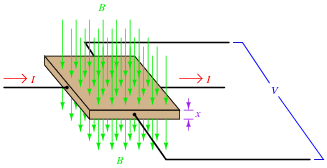# Learning Electronics

Learn to build electronic circuits

# DC transducers

### Question 1:

Research and describe what the Hall effect is, and list some practical uses of it.
There are many good references describing the nature and discovery of the Hall effect. I'll let you find this on your own!
Practical applications of the Hall effect include magnetic field measurement, non-contact current measurement, position sensors, and analog computation.

Notes:
One of the most interesting applications of the Hall effect I've ever seen was an analog multiplier, which output a voltage signal proportional to the product of two input signals. In the specific example I saw, the two input signals were voltage and current for an AC load, the output of the Hall element representing instantaneous load power. A precise fraction of the AC load current passed through the Hall element, while a precise fraction of the AC load voltage energized a coil to generate a magnetic field flux perpendicular to the current (through the Hall element).

### Question 2:

The following equation is a general model for Hall effect devices, describing the voltage produced by a Hall element given the current through it (I), the magnetic flux density (B) passing through perpendicular to the current, and the thickness (x) of the Hall element. The variable K is a constant accounting for variations in material composition and temperature, and for any combination of units represented by the other variables:

 VHall = K IB xExplain what this equation means with regard to the effect of each variable (I, B, and x) on the Hall voltage generated. Identify whether each of the variables has a direct or an inverse effect on the output voltage.
I = direct effect
B = direct effect
x = inverse effect

Notes:
Discuss with your students what it means for a variable to have a direct or inverse effect on another, and ask them to provide examples other than the Hall effect equation. If they cannot think of any, suggest Ohm's Law.